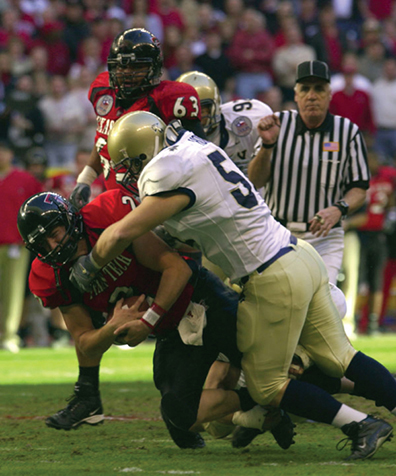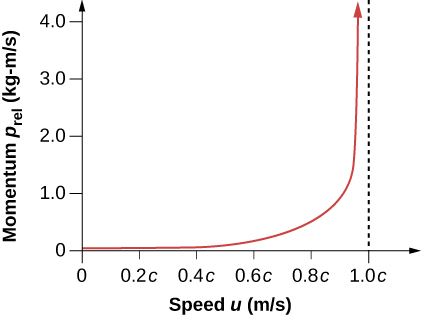# 5.8 Relativistic momentum

 Page 1 / 3
By the end of this section, you will be able to:
• Define relativistic momentum in terms of mass and velocity
• Show how relativistic momentum relates to classical momentum
• Show how conservation of relativistic momentum limits objects with mass to speeds less than $c$

Momentum is a central concept in physics. The broadest form of Newton’s second law is stated in terms of momentum. Momentum is conserved whenever the net external force on a system is zero. This makes momentum conservation a fundamental tool for analyzing collisions ( [link] ). Much of what we know about subatomic structure comes from the analysis of collisions of accelerator-produced relativistic particles, and momentum conservation plays a crucial role in this analysis.Momentum is an important concept for these football players from the University of California at Berkeley and the University of California at Davis. A player with the same velocity but greater mass collides with greater impact because his momentum is greater. For objects moving at relativistic speeds, the effect is even greater.

The first postulate of relativity states that the laws of physics are the same in all inertial frames. Does the law of conservation of momentum survive this requirement at high velocities? It can be shown that the momentum calculated as merely $\stackrel{\to }{p}=m\frac{d\stackrel{\to }{x}}{dt},$ even if it is conserved in one frame of reference, may not be conserved in another after applying the Lorentz transformation to the velocities. The correct equation for momentum can be shown, instead, to be the classical expression in terms of the increment $d\tau$ of proper time of the particle, observed in the particle’s rest frame:

$\begin{array}{cc}\hfill \stackrel{\to }{p}& =m\frac{d\stackrel{\to }{x}}{d\tau }=m\frac{d\stackrel{\to }{x}}{dt}\phantom{\rule{0.1em}{0ex}}\frac{dt}{d\tau }\hfill \\ & =m\frac{d\stackrel{\to }{x}}{dt}\phantom{\rule{0.2em}{0ex}}\frac{1}{\sqrt{1-{u}^{2}\text{/}{c}^{2}}}\hfill \\ & =\frac{m\stackrel{\to }{u}}{\sqrt{1-{u}^{2}\text{/}{c}^{2}}}=\gamma m\stackrel{\to }{u}.\hfill \end{array}$

## Relativistic momentum

Relativistic momentum $\stackrel{\to }{p}$ is classical momentum multiplied by the relativistic factor γ :

$\stackrel{\to }{p}=\gamma m\stackrel{\to }{u}$

where m is the rest mass    of the object, $\stackrel{\to }{u}$ is its velocity relative to an observer, and γ is the relativistic factor:

$\gamma =\frac{1}{\sqrt{1-\frac{{u}^{2}}{{c}^{2}}}}.$

Note that we use u for velocity here to distinguish it from relative velocity v between observers. The factor $\gamma$ that occurs here has the same form as the previous relativistic factor $\gamma$ except that it is now in terms of the velocity of the particle u instead of the relative velocity v of two frames of reference.

With p expressed in this way, total momentum ${p}_{\text{tot}}$ is conserved whenever the net external force is zero, just as in classical physics. Again we see that the relativistic quantity becomes virtually the same as the classical quantity at low velocities, where u / c is small and $\gamma$ is very nearly equal to 1. Relativistic momentum has the same intuitive role as classical momentum. It is greatest for large masses moving at high velocities, but because of the factor $\gamma ,$ relativistic momentum approaches infinity as u approaches c ( [link] ). This is another indication that an object with mass cannot reach the speed of light. If it did, its momentum would become infinite—an unreasonable value.Relativistic momentum approaches infinity as the velocity of an object approaches the speed of light.

The relativistically correct definition of momentum as $p=\gamma mu$ is sometimes taken to imply that mass varies with velocity: ${m}_{\text{var}}=\gamma m,$ particularly in older textbooks. However, note that m is the mass of the object as measured by a person at rest relative to the object. Thus, m is defined to be the rest mass, which could be measured at rest, perhaps using gravity. When a mass is moving relative to an observer, the only way that its mass can be determined is through collisions or other means involving momentum. Because the mass of a moving object cannot be determined independently of momentum, the only meaningful mass is rest mass. Therefore, when we use the term “mass,” assume it to be identical to “rest mass.”

Relativistic momentum is defined in such a way that conservation of momentum holds in all inertial frames. Whenever the net external force on a system is zero, relativistic momentum is conserved, just as is the case for classical momentum. This has been verified in numerous experiments.

Check Your Understanding What is the momentum of an electron traveling at a speed 0.985 c ? The rest mass of the electron is $9.11\phantom{\rule{0.2em}{0ex}}×\phantom{\rule{0.2em}{0ex}}{10}^{-31}\text{kg}.$

Substitute the data into the given equation:
$p=\gamma mu=\frac{mu}{\sqrt{1-\frac{{u}^{2}}{{c}^{2}}}}=\frac{\left(9.11\phantom{\rule{0.2em}{0ex}}×\phantom{\rule{0.2em}{0ex}}{10}^{-31}\phantom{\rule{0.2em}{0ex}}\text{kg}\right)\left(0.985\right)\left(3.00\phantom{\rule{0.2em}{0ex}}×\phantom{\rule{0.2em}{0ex}}{10}^{8}\phantom{\rule{0.2em}{0ex}}\text{m/s}\right)}{\sqrt{1-\frac{{\left(0.985c\right)}^{2}}{{c}^{2}}}}=1.56\phantom{\rule{0.2em}{0ex}}×\phantom{\rule{0.2em}{0ex}}{10}^{-21}\phantom{\rule{0.2em}{0ex}}\text{kg-m/s.}$

## Summary

• The law of conservation of momentum is valid for relativistic momentum whenever the net external force is zero. The relativistic momentum is $p=\gamma mu,$ where m is the rest mass of the object, u is its velocity relative to an observer, and the relativistic factor is $\gamma =\frac{1}{\sqrt{1-\frac{{u}^{2}}{{c}^{2}}}}.$
• At low velocities, relativistic momentum is equivalent to classical momentum.
• Relativistic momentum approaches infinity as u approaches c . This implies that an object with mass cannot reach the speed of light.

## Conceptual questions

How does modern relativity modify the law of conservation of momentum?

Is it possible for an external force to be acting on a system and relativistic momentum to be conserved? Explain.

Yes. This can happen if the external force is balanced by other externally applied forces, so that the net external force is zero.

## Problems

Find the momentum of a helium nucleus having a mass of $6.68\phantom{\rule{0.2em}{0ex}}×\phantom{\rule{0.2em}{0ex}}{10}^{-27}\phantom{\rule{0.2em}{0ex}}\text{kg}$ that is moving at 0.200 c .

$4.09\phantom{\rule{0.2em}{0ex}}×\phantom{\rule{0.2em}{0ex}}{10}^{-19}\phantom{\rule{0.2em}{0ex}}\text{kg}·\text{m/s}$

What is the momentum of an electron traveling at 0.980 c ?

(a) Find the momentum of a $1.00\phantom{\rule{0.2em}{0ex}}×\phantom{\rule{0.2em}{0ex}}{10}^{9}\text{-kg}$ asteroid heading towards Earth at 30.0 km/s. (b) Find the ratio of this momentum to the classical momentum. (Hint: Use the approximation that $\gamma =1+\left(1\text{/}2\right){v}^{2}\text{/}{c}^{2}$ at low velocities.)

a. $3.000000015\phantom{\rule{0.2em}{0ex}}×\phantom{\rule{0.2em}{0ex}}{10}^{13}\phantom{\rule{0.2em}{0ex}}\text{kg}·\text{m/s;}$ b. 1.000000005

(a) What is the momentum of a 2000-kg satellite orbiting at 4.00 km/s? (b) Find the ratio of this momentum to the classical momentum. (Hint: Use the approximation that $\gamma =1+\left(1\text{/}2\right){v}^{2}\text{/}{c}^{2}$ at low velocities.)

What is the velocity of an electron that has a momentum of $3.04\phantom{\rule{0.2em}{0ex}}×\phantom{\rule{0.2em}{0ex}}{10}^{-21}\phantom{\rule{0.2em}{0ex}}\text{kg}·\text{m/s}$ ? Note that you must calculate the velocity to at least four digits to see the difference from c .

$2.988\phantom{\rule{0.2em}{0ex}}×\phantom{\rule{0.2em}{0ex}}{10}^{8}\phantom{\rule{0.2em}{0ex}}\text{m/s}$

Find the velocity of a proton that has a momentum of $4.48\phantom{\rule{0.2em}{0ex}}×\phantom{\rule{0.2em}{0ex}}{10}^{-19}\phantom{\rule{0.2em}{0ex}}\text{kg}·\text{m/s}\text{.}$

fundamental note of a vibrating string
what are matter waves? Give some examples
according to de Broglie any matter particles by attaining the higher velocity as compared to light'ill show the wave nature and equation of wave will applicable on it but in practical life people see it is impossible however it is practicaly true and possible while looking at the earth matter at far
Manikant
Mathematical expression of principle of relativity
given that the velocity v of wave depends on the tension f in the spring, it's length 'I' and it's mass 'm'. derive using dimension the equation of the wave
What is the importance of de-broglie's wavelength?
he related wave to matter
Zahid
at subatomic level wave and matter are associated. this refering to mass energy equivalence
Zahid
it is key of quantum
Manikant
how those weight effect a stable motion at equilibrium
how do I differentiate this equation- A sinwt with respect to t
just use the chain rule : let u =wt , the dy/dt = dy/du × du/dt : wA × cos(wt)
Jerry
I see my message got garbled , anyway use the chain rule with u= wt , etc...
Jerry
de broglie wave equation
vy beautiful equation
chandrasekhar
what is electro statics
when you consider systems consisting of fixed charges
Sherly
Diagram of the derive rotational analog equation of v= u+at
what is carat
a unit of weight for precious stones and pearls, now equivalent to 200 milligrams.
LoNE
a science that deals with the composition, structure, and properties of substances and with the transformations that they undergo.
LoNE
what is chemistry
what chemistry ?
Abakar
where are the mcq
ok
Giorgi
acids and bases
Navya
How does unpolarized light have electric vector randomly oriented in all directions.
unpolarized light refers to a wave collection which has an equal distribution of electric field orientations for all directions
pro
In a grating, the angle of diffraction for second order maximum is 30°.When light of wavelength 5*10^-10cm is used. Calculate the number of lines per cm of the grating.
OK I can solve that for you using Bragg's equation 2dsin0over lander
ossyBy OpenStaxBy Jazzycazz JacksonBy Savannah ParrishBy John GabrieliBy Dionne MahaffeyBy Janet ForresterBy Darlene PaliswatBy Cath YuBy Christine ZeelieBy Kimberly Nichols